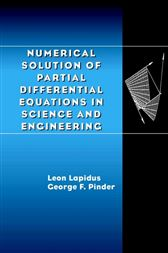Numerical Solution of Partial Differential Equations in Science and EngineeringUS\$208.00
US\$187.20
ISBNs
• 1118031210
• 9780471098669
• 9781118031216
From the reviews of Numerical Solution of Partial Differential Equations in Science and Engineering:

"The book by Lapidus and Pinder is a very comprehensive, even exhaustive, survey of the subject . . . [It] is unique in that it covers equally finite difference and finite element methods."
Burrelle's

"The authors have selected an elementary (but not simplistic) mode of presentation. Many different computational schemes are described in great detail . . . Numerous practical examples and applications are described from beginning to the end, often with calculated results given."
Mathematics of Computing

"This volume . . . devotes its considerable number of pages to lucid developments of the methods [for solving partial differential equations] . . . the writing is very polished and I found it a pleasure to read!"
Mathematics of Computation

Of related interest . . .
NUMERICAL ANALYSIS FOR APPLIED SCIENCE Myron B. Allen and Eli L. Isaacson. A modern, practical look at numerical analysis, this book guides readers through a broad selection of numerical methods, implementation, and basic theoretical results, with an emphasis on methods used in scientific computation involving differential equations. 1997 (0-471-55266-6) 512 pp.

APPLIED MATHEMATICS Second Edition, J. David Logan. Presenting an easily accessible treatment of mathematical methods for scientists and engineers, this acclaimed work covers fluid mechanics and calculus of variations as well as more modern methods-dimensional analysis and scaling, nonlinear wave propagation, bifurcation, and singular perturbation. 1996 (0-471-16513-1) 496 pp.

• Wiley; February 2011
• ISBN: 9781118031216
• Edition: 1
• Title: Numerical Solution of Partial Differential Equations in Science and Engineering
• Author: Leon Lapidus; George F. Pinder
• Imprint: Wiley-Interscience
ISBNs
• 1118031210
• 9780471098669
• 9781118031216